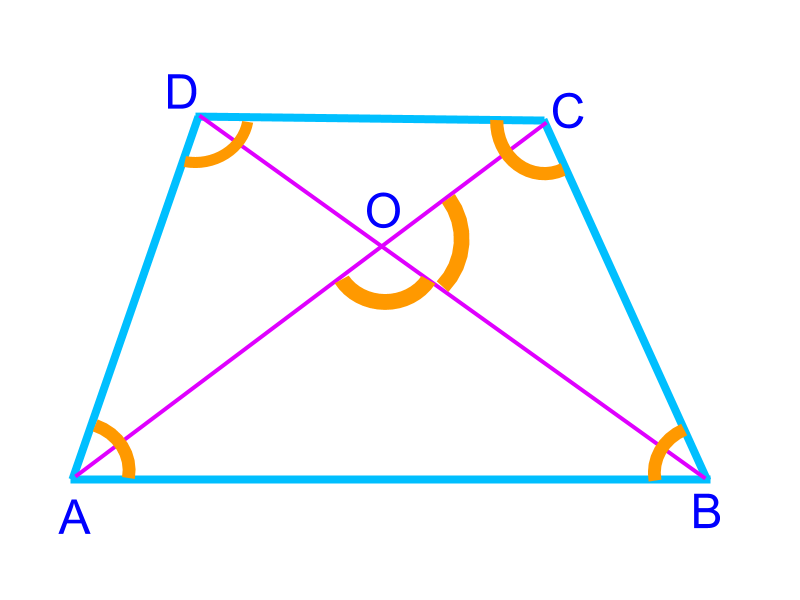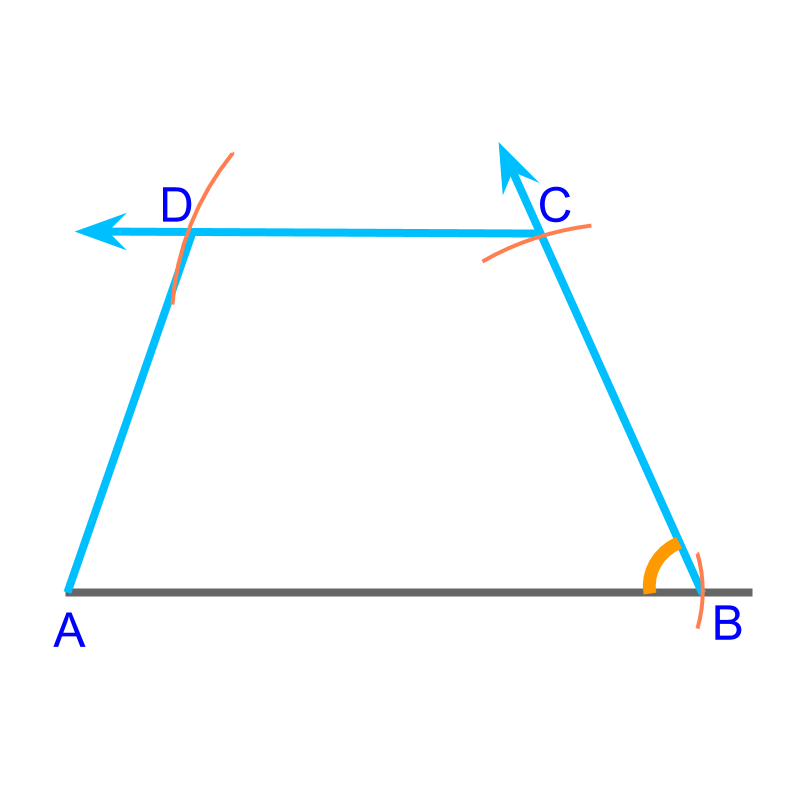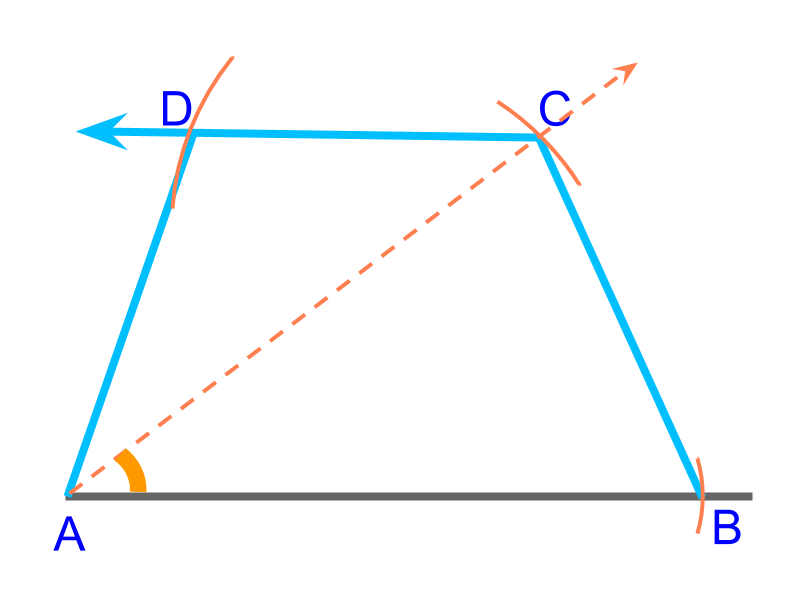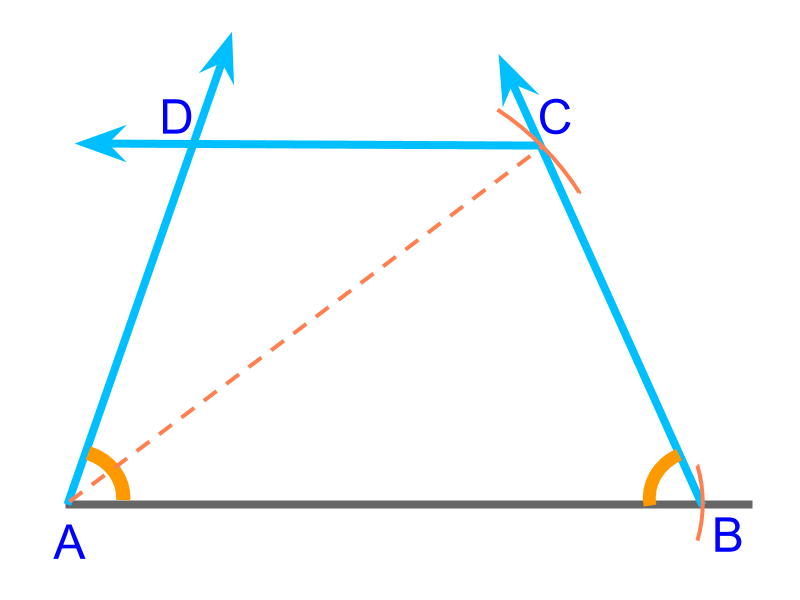maths > construction-basics

Construction of Trapezium

what you'll learn...

overview

In this page, constructing trapezium is explained. It is outlined as follows.

•  Properties of trapezium is explained

•  The number of independent parameters in a trapezium is 4$4$

•  For a given parameter, construction of trapezium is approached as combination of triangles (sss, sas, asa, rhs, sal) and using the properties of trapezium.

Understanding Trapezium

A trapezium is a quadrilateral with one pair of parallel sides.Quadrilateral is defined by 5$5$ parameters. In a trapezium, the following property provides dependence of parameters

•  one pair of sides are parallel

Note: The sides that are parallel are called bases. The other two sides are referred as sides.

A trapezium is defined by 4$4$ parameters.To construct a trapezium, 2$2$ bases (¯¯¯¯¯¯AB$\overline{A B}$, ¯¯¯¯¯¯CD$\overline{C D}$) , $1$ diagonal ($\overline{A C}$) and $1$ side ($\overline{B C}$) are given. This is illustrated in the figure. To construct, consider as an SSS triangle in $A B C$ and mark $D$ on a parallel.To construct a trapezium, $2$ bases ($\overline{A B}$, $\overline{C D}$), $1$ side ($\overline{B C}$), and an angle ($\angle B$) are given. This is illustrated in the figure. To construct, consider this as a SAS triangle in $A B C$ and mark $D$ on a parallelTo construct a trapezium, $2$ bases ($\overline{A B}$, $\overline{C D}$), a diagonal ($\overline{A C}$), and the angle between diagonal and the base ($\angle C A B$)are given. This is illustrated in the figure. To construct, consider this as a SAS triangle in $C A B$ and mark $D$ on a parallel.To construct a trapezium, a base($\overline{A B}$), a diagonal ($\overline{A C}$), and $2$ angles ($\angle A$, $\angle B$) on the given base are given. This is illustrated in the figure. To construct, consider this as a SAS triangle in $A B C$ and construct ray $A D$ to mark $D$ on a parallel

summaryConstruction of Trapezium :

Properties of trapezium:

•  one pair of sides are parallel

Note: The sides that are parallel are called bases. The other two sides are referred as sides.

The formulations of questions

•  2 bases, 1 side, 1 diagonal

•  2 bases, 1 diagonal, 1 angle between one base and the given diagonal

•  2 bases, 1 side, 1 angle between one base and given side

•  1 base, 2 angles on the given base, 1 diagonal

use properties to figure out dependent parameters and look for triangles.

Outline